# MCAT Physical : Laws of Thermodynamics

## Example Questions

### Example Question #41 : Biochemistry, Organic Chemistry, And Other Concepts

A student conducts an experiment for a chemistry class. The student wishes to explore power generation from different types of voltaic cells. He sets up three different cells, and then compares the amount of energy generated from each one.

One of his cells is shown below as an example. Both remaining cells follow the same layout.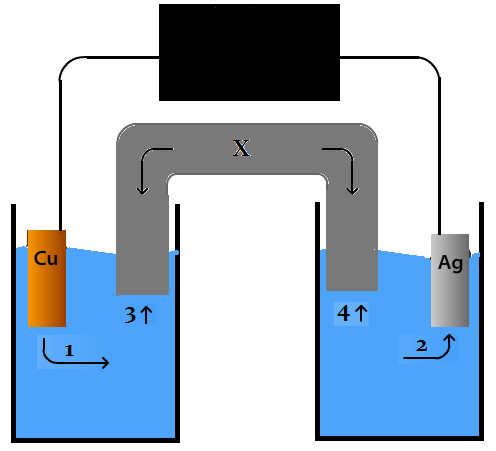A scientist discovers that a voltaic cell he constructs is able to run and produce energy indefinitely, without ever needing intervention again. Which law is this cell violating?

The second law of thermodynamics

The cell is not violating thermodynamics

The zeroth law of thermodynamics

The first law of thermodynamics

The third law of thermodynamics

The first law of thermodynamics

Explanation:

The first law of thermodynamics is the conservation of energy law. A cell that produces energy indefinitely is functionally a perpetual motion machine, and is prohibited by the first law.

### Example Question #42 : Biochemistry, Organic Chemistry, And Other Concepts

A researcher measures the kinetic energy of a chair sliding on a rough floor. At the beginning of motion the chair has a kinetic energy of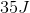. Once the chair stops sliding the kinetic energy is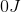. What can you conclude about the researcher’s results?

The results are invalid because they violate the first law of thermodynamics

The results do not violate the second law of thermodynamics because energy can be destroyed if entropy increases

The results do not violate the first law of thermodynamics because energy is not destroyed

The results are invalid because they violate the second law of thermodynamics

The results do not violate the first law of thermodynamics because energy is not destroyed

Explanation:

The first law of thermodynamics states that the energy of the universe is constant because energy cannot be created or destroyed; however, it is possible for energy to be converted from one form to another. In this question the chair has kinetic energy in the beginning, but it loses all of this energy once it stops. This doesn’t mean that the kinetic energy is destroyed; it means that the kinetic energy is converted to another form of energy. Since it is sliding on a floor with a lot of friction (rough floor), the chair converts the kinetic energy to heat.

The second law of thermodynamics states that the entropy of the universe is always increasing. This law is irrelevant to the question.

### Example Question #43 : Biochemistry, Organic Chemistry, And Other Concepts

Which of the following is true of an adiabatic expansion?

Temperature increases

Heat is gained

Temperature remains constant

Heat is lost

Temperature decreases

Temperature decreases

Explanation:

In thermodynamics, no heat is exchanged in an adiabatic process, so heat is neither gained, nor lost. In an adiabatic compression temperature increases, but in an expansion temperature decreases.

### Example Question #1 : Laws Of Thermodynamics

A scientist prepares an experiment to demonstrate the second law of thermodynamics for a chemistry class. In order to conduct the experiment, the scientist brings the class outside in January and gathers a cup of water and a portable stove.

The temperature outside is –10 degrees Celsius. The scientist asks the students to consider the following when answering his questions:

Gibbs Free Energy Formula:

ΔG = ΔH – TΔS

Liquid-Solid Water Phase Change Reaction:

H2O(l) ⇌ H2O(s) + X

The scientist prepares two scenarios.

Scenario 1:

The scientist buries the cup of water outside in the snow, returns to the classroom with his class for one hour, and the class then checks on the cup. They find that the water has frozen in the cup.

Scenario 2:

The scientist then places the frozen cup of water on the stove and starts the gas. The class finds that the water melts quickly.

After the water melts, the scientist asks the students to consider two hypothetical scenarios as a thought experiment.

Scenario 3:

Once the liquid water at the end of scenario 2 melts completely, the scientist turns off the gas and monitors what happens to the water. Despite being in the cold air, the water never freezes.

Scenario 4:

The scientist takes the frozen water from the end of scenario 1, puts it on the active stove, and the water remains frozen.

The same scientist in the passage measures the variables of another reaction in the lab. He knows that this reaction is spontaneous under standard conditions, with a standard free energy change of –43 kJ/mol. Using laboratory-calculated variables, he determines that the Gibbs Free Energy has a value of 0 kJ/mol. What can we say about this reaction?

The scientist made an error, all spontaneous reactions have a negative Gibbs Free Energy value.

43 kJ of energy must be put into the system to have the reaction proceed spontaneously.

It indicates that the reaction will produce 43 kJ of energy for every mole that is allowed to react spontaneously under current conditions.

The reaction will always produce 43 kJ of heat when it reacts to completion.

Explanation:

A reaction with a zero free energy change is at equilibrium. At standard conditions, not at equilibrium, this reaction would have a Gibbs free energy change of –43 kJ/mol, would be spontaneous, and would be able to produce 43 kJ of useful work for every mole that reacts.

### Example Question #2 : Laws Of Thermodynamics

An air conditioning unit is supplied with power to effectively cool an entire house without generating heat.

Which law(s) of thermodynamics is(are) violated?

I. The first law

II. The second law

III. The third law

II only

I and II

III only

I only

II and III

II only

Explanation:

The first law of thermodynamics, conservation of energy, is not violated since the refrigerator is merely transforming electrical energy into work.

The second law of thermodynamics, increase of entropy, is violated since the entropy of a cooled house is lower, but there is no corresponding increase in entropy elsewhere. In order to cool an object in the system, and object in the surroundings must be heated.

The third law of thermodynamics deals with absolute zero and is not relevant.

### Example Question #3 : Laws Of Thermodynamics

Consider the following reaction.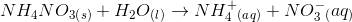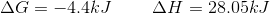This reaction is endergonic because the enthalpy change is greater than zero

This reaction increases the entropy of the universe because it is a non-spontaneous reaction

This reaction increases the entropy of the universe because it is a spontaneous reaction

This reaction is exothermic because the free energy change is less than zero

This reaction increases the entropy of the universe because it is a spontaneous reaction

Explanation:

The question states that the change in Gibbs free energy,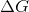, is negative. This means that the reaction is spontaneous. Second law of thermodynamics states that a spontaneous process always increases the entropy of the universe. Note that the second law only addresses the entropy of the universe; the change in entropy of a reaction could be either positive or negative.

This reaction is exergonic (spontaneous) because the change in Gibbs free energy () is negative. It is also endothermic because the change in enthalpy (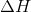) is positive.

### Example Question #4 : Laws Of Thermodynamics

Which of the following will result in heat transfer?

Enthalpy increases

Entropy increases

Internal energy is stable

Explanation:

Heat is a form of energy resulting from molecular velocity. Temperature is a scale to measure the amount of internal energy stored as heat, known as enthalpy. Entropy is the relative disorder of a system.

In order for a heat transfer to occur, the system must not be in equilibrium. In other words, there must be a difference in heat energy between two regions of the system, which will allow heat energy to travel from the region of high energy to the region of low energy. This condition is easily identified by a temperature gradient, in which heat will from the region of high temperature to the region of low temperature until the system is at an equilibrium temperature.

### Example Question #5 : Laws Of Thermodynamics

Which of the following is an implication of the zeroth law of thermodynamics?

Entropy of the universe is always increasing

Energy in the universe cannot be created or destroyed

If systems A and B are in thermal equilibrium with system C, then systems A, B, and C are in thermal equilibrium with each other

A pure crystal kept at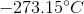has a very low entropy

If systems A and B are in thermal equilibrium with system C, then systems A, B, and C are in thermal equilibrium with each other

Explanation:

This question asks about the zeroth law of thermodynamics which states if two systems are in thermal equilibrium with another system, then all three systems will be in thermal equilibrium with each other.

The other answer choices in this question talk about the other three laws of thermodynamics. First law of thermodynamics states that energy of the universe is constant and that energy cannot be created or destroyed. The second law states that the entropy of the universe is constantly increasing, even if local entropy decreases. The third law states that a pure crystal at absolute zero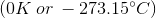has zero entropy.

### Example Question #6 : Laws Of Thermodynamics

Two children are playing on an icy lake. Child 1 weighs 50kg, and child 2 weighs 38kg. Child 1 has a backpack that weighs 10kg, and child 2 has a backpack that weighs 5kg.

Over the course of the afternoon, they collide many times. Four collisions are described below.

Collision 1:

Child 1 starts from the top of a ramp, and after going down, reaches the lake surface while going 5m/s and subsequently slides into a stationary child 2. They remain linked together after the collision.

Collision 2:

Child 1 and child 2 are sliding in the same direction. Child 2, moving at 10m/s, slides into child 1, moving at 2m/s.

Collision 3:

The two children collide while traveling in opposite directions at 10m/s each.

Collision 4:

The two children push off from one another’s back, and begin moving in exactly opposite directions. Child 2 moves with a velocity of +8m/s.

In collision 1, child 1 stops moving under the opposing force of friction. After she stops moving, the energy she lost to the lake as heat, via friction, is exactly equal to the amount of kinetic energy she lost from the moment she struck the lake surface to when she stopped. What law prevents this lost heat energy from ever moving the child again?

Zeroth Law of Thermodynamics

First Law of Thermodynamics

The principle that entropy of the universe always decreases

Second Law of Thermodynamics

Third Law of Thermodynamics